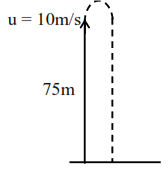# A balloon was moving upwards`
Question:

A balloon was moving upwards with a uniform velocity of $10 \mathrm{~m} / \mathrm{s}$. An object of finite mass is dropped from the balloon when it was at a height of $75 \mathrm{~m}$ from the ground level. The height of the balloon from the ground when object strikes the ground was around :

(takes the value of $g$ as $10 \mathrm{~m} / \mathrm{s}^{2}$ )

1. $300 \mathrm{~m}$

2. $200 \mathrm{~m}$

3. $125 \mathrm{~m}$

4. $250 \mathrm{~m}$

Correct Option: , 3

Solution:Object is projected as shown so as per motion under gravity

$S=u t+\frac{1}{2} a t^{2}$

$-75=+10 t+\frac{1}{2}(-10) t^{2} \Rightarrow t=5 \mathrm{sec}$

Object takes $t=5 \mathrm{~s}$ to fall on ground

Height of balloon from ground

$\mathrm{H}=75+\mathrm{ut}$

$=75+10 \times 5=125 \mathrm{~m}$